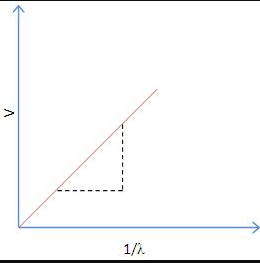# how to calculate planck's constant from a graph

The slope of a plot of frequency and energy of a photon gives Planck's constant.

To calculate Planck's constant, plot the energy of the photon on the y-axis in units of Joules and frequency of the wave on the x-axis in units of Hertz (sec−1). The calculate the slope y2-y1/x2-x1 which should equal Planck's constant at 6.626 x 10−34 Joule seconds (Js).• 2
What are you looking for?